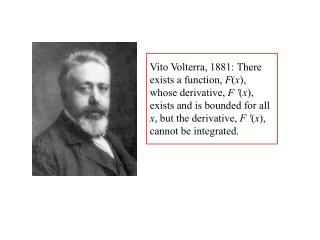DownloadDownload PresentationThe Fundamental Theorem of Calculus:

# The Fundamental Theorem of Calculus:

Télécharger la présentation## The Fundamental Theorem of Calculus:

- - - - - - - - - - - - - - - - - - - - - - - - - - - E N D - - - - - - - - - - - - - - - - - - - - - - - - - - -
##### Presentation Transcript

1. Vito Volterra, 1881: There exists a function, F(x), whose derivative, F '(x), exists and is bounded for all x, but the derivative, F '(x), cannot be integrated.

2. The Fundamental Theorem of Calculus: 1. If then 2.If f does not have a jump discontinuity at x, then

3. If F is differentiable at x = a, can F '(x) be discontinuous at x = a?

4. Yes! If F is differentiable at x = a, can F '(x) be discontinuous at x = a?

5. “Cantor’s Set” First described by H.J.S. Smith, 1875

6. “Cantor’s Set” First described by H.J.S. Smith, 1875 Then by Vito Volterra, 1881

7. “Cantor’s Set” First described by H.J.S. Smith, 1875 Then by Vito Volterra, 1881 And finally by Georg Cantor, 1883

8. Cantor’s Set 2/3 1 0 1/3 Remove [1/3,2/3]

9. Cantor’s Set 1/9 2/9 7/9 8/9 0 1/3 2/3 1 Remove [1/3,2/3] Remove [1/9,2/9] and [7/9,8/9]

10. Cantor’s Set 1/9 2/9 7/9 8/9 0 1/3 2/3 1 Remove [1/3,2/3] Remove [1/9,2/9] and [7/9,8/9] Remove [1/27,2/27], [7/27,8/27], [19/27,20/27], and [25/27,26/27], and so on … What’s left?

11. Cantor’s Set 1/9 2/9 7/9 8/9 0 1/3 2/3 1 What’s left has measure 0.

12. Create a new set like the Cantor set except the first middle piece only has length 1/4 each of the next two middle pieces only have length 1/16 the next four pieces each have length 1/64, etc. The amount left has size

13. We’ll call this set SVC (for Smith-Volterra-Cantor). It has some surprising characteristics: 1. SVC contains no intervals - no matter how small a subinterval of [0,1] we take, there will be points in that subinterval that are not in SVC. SVC is nowhere dense.

14. We’ll call this set SVC (for Smith-Volterra-Cantor). It has some surprising characteristics: 1. SVC contains no intervals - no matter how small a subinterval of [0,1] we take, there will be points in that subinterval that are not in SVC. SVC is nowhere dense. 2. Given any collection of subintervals of [0,1], whose union contains SVC, the sum of the lengths of these intervals is at least 1/2.

15. Volterra’s construction: Start with the function Restrict to the interval [0,1/8], except find the largest value of x on this interval at which F '(x) = 0, and keep F constant from this value all the way to x = 1/8.

16. Volterra’s construction: To the right of x = 1/8, take the mirror image of this function: for 1/8 < x < 1/4, and outsideof [0,1/4], define this function to be 0. Call this function .

17. Volterra’s construction: is a differentiable function for all values of x, but

18. Now we slide this function over so that the portion that is not identically 0 is in the interval [3/8,5/8], that middle piece of length 1/4 taken out of the SVC set.

19. We follow the same procedure to create a new function, , that occupies the interval [0,1/16] and is 0 outside this interval.

20. We slide one copy of into each interval of length 1/16 that was removed from the SVC set.

21. Volterra’s function, V(x), is what we obtain in the limit as we do this for every interval removed from the SVC set. It has the following properties: 1. V is differentiable at every value of x, and its derivative is bounded (below by –1.013 and above by 1.023).

22. Volterra’s function, V(x), is what we obtain in the limit as we do this for every interval removed from the SVC set. It has the following properties: 1. V is differentiable at every value of x, and its derivative is bounded (below by –1.013 and above by 1.023). 2. If a is a left or right endpoint of one of the removed intervals, then the derivative of V at a exists (and equals 0), but we can find points arbitrarily close to a where the derivative is +1, and points arbitrarily close to a where the derivative is –1.

23. No matter how we partition [0,1], the pieces that contain endpoints of removed intervals must have lengths that add up to at least 1/2. The pieces on which the variation of V ' is at least 2 must have lengths that add up to at least 1/2.

24. Recall: Integral exists if and only if can be made as small as we wish by taking sufficiently small intervals.

25. Conclusion: Volterra’s function V can be differentiated and has a bounded derivative, but its derivative, V ', cannot be integrated: